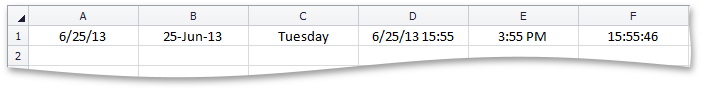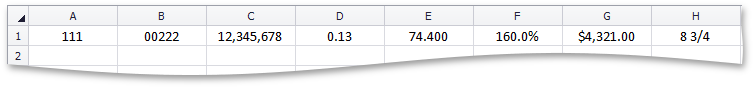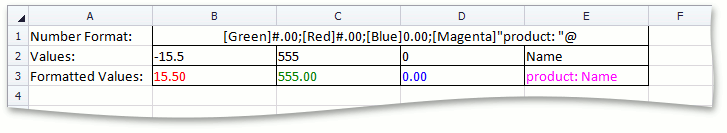21.1
20.2
20.1
19.2
19.1
18.2
18.1
17.2
A newer version of this page is available. Switch to the current version.

# How to: Specify Number or Date Format for Cell Content

• 3 minutes to read

You can specify how to display numeric values in cells by applying number formats. For example, a number can appear in a cell as a percentage, decimal, currency, accounting, date or time value. To apply a number format to a cell or cell range, assign the corresponding number format code to the Formatting.NumberFormat property of the cell or cell range object. This document provides examples on how to apply different number formats to display cell content as date and time and as percentage, currency and decimal values, and how to create custom number formats.

## Date and Time Formats

This example demonstrates how to specify different display formats for date and time values in cells.

##### NOTE

A complete sample project is available at https://github.com/DevExpress-Examples/winforms-spreadsheetcontrol-api-e4655

``````worksheet.Range["A1:F1"].Formula = "= Now()";

// Apply different date display formats.
worksheet.Cells["A1"].NumberFormat = "m/d/yy";

worksheet.Cells["B1"].NumberFormat = "d-mmm-yy";

worksheet.Cells["C1"].NumberFormat = "dddd";

// Apply different time display formats.
worksheet.Cells["D1"].NumberFormat = "m/d/yy h:mm";

worksheet.Cells["E1"].NumberFormat = "h:mm AM/PM";

worksheet.Cells["F1"].NumberFormat = "h:mm:ss";
``````

The image below shows the different formats used to display date and time values in cells.## Number Formats

This example demonstrates how to use different number formats to display numeric data using the most appropriate formats.

##### NOTE

A complete sample project is available at https://github.com/DevExpress-Examples/winforms-spreadsheetcontrol-api-e4655

``````// Display 111 as 111.
worksheet.Cells["A1"].Value = 111;
worksheet.Cells["A1"].NumberFormat = "#####";

// Display 222 as 00222.
worksheet.Cells["B1"].Value = 222;
worksheet.Cells["B1"].NumberFormat = "00000";

// Display 12345678 as 12,345,678.
worksheet.Cells["C1"].Value = 12345678;
worksheet.Cells["C1"].NumberFormat = "#,#";

// Display .126 as 0.13.
worksheet.Cells["D1"].Value = .126;
worksheet.Cells["D1"].NumberFormat = "0.##";

// Display 74.4 as 74.400.
worksheet.Cells["E1"].Value = 74.4;
worksheet.Cells["E1"].NumberFormat = "##.000";

// Display 1.6 as 160.0%.
worksheet.Cells["F1"].Value = 1.6;
worksheet.Cells["F1"].NumberFormat = "0.0%";

// Display 4321 as \$4,321.00.
worksheet.Cells["G1"].Value = 4321;
worksheet.Cells["G1"].NumberFormat = "\$#,##0.00";

// Display 8.75 as 8 3/4.
worksheet.Cells["H1"].Value = 8.75;
worksheet.Cells["H1"].NumberFormat = "# ?/?";
``````

The image below shows the different formats used to display numbers in cells.## Custom Number Formats

This example demonstrates how to create a custom number format to display positive numbers, negative numbers, zero and text values using different formats and colors. To do this, specify the appropriate code sections of the number format as required. These code sections are separated by semicolons and used in the following order.

<POSITIVE>;<NEGATIVE>;<ZERO>;<TEXT>

##### NOTE

A complete sample project is available at https://github.com/DevExpress-Examples/winforms-spreadsheetcontrol-api-e4655

``````// Set cell values.
worksheet["B2:B3"].Value = -15.50;
worksheet["C2:C3"].Value = 555;
worksheet["D2:D3"].Value = 0;
worksheet["E2:E3"].Value = "Name";

//Apply custom number format.
worksheet["B3:E3"].NumberFormat = "[Green]#.00;[Red]#.00;[Blue]0.00;[Magenta]\"product: \"@";
``````

The image below shows how different values are displayed in cells when a custom number format is applied.See Also